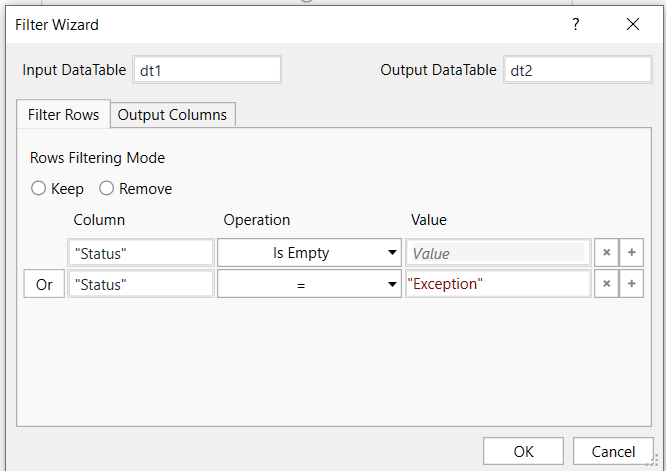# I want to check 2 conditions in if condition

i want to check 2 conditions in Data table in one single if condition activity like row(“Status”)=“” and row(“Status”)=“Exception”

condition should check both the criteria in data table and then it should move

plz provide the solution

You can try the three ways

1. using if condition:

Steps to be followed

a. Read the excel and store in dt1

b. use for each row to loop through each row of dt1

iniside the for each row , use the if condition with condition as

row(“Status”).ToString=“” or row(“Status”).ToString=“Exception”

then do accordingly in the else and then section

OR

1. Use Filter datatable activivty

Steps to be followed

a. Read the excel and store in dt1

b. Use filter datatable activvity with below property values as in below imageOR

1. Linq query

Steps to be followed

a. Read the excel and store in dt1
b. use assign activity

dt2 = dt1.AsEnumerable().Where(Function(row) row(“Status”).ToString.Equals(“”) Or row(“Status”).ToString.Equals(“Exception”)).CopyToDataTable

c. this will output with row having status null or exception

Hope it helps you

Mark it as solution if resolves your query

Regards
Nived N
Happy Automation

row(“Status”)=“” and row(“Status”)=“Exception” . de we have to use .tostring here

Yes u need use that too